# Peltier Elements

Peltier elements / thermoelectric coolers (TECs) are heat pumps which transfer heat from one side to the other, depending on the direction of the electrical current. TEC controllers are used to drive the Peltier elements.
This article explains how Peltier elements / thermoelectric coolers work, describes the features and mentions manufacturers of Peltier elements.

TEC Controller Product Overview

Contents

## Basics of Peltier Element

A Peltier element is able to transport heat using the Peltier effect. Inside the Peltier element the Peltier effect produces a temperature difference between two side when a current is flowing.

Depending on the direction of the DC current flow it's possible to cool and heat with Peltier elements without changing the connectors or mechanical setup. Further advantages are that small designs can be realized and there are no moving parts. The current supplied to the Peltier element is controlled by a TEC controller.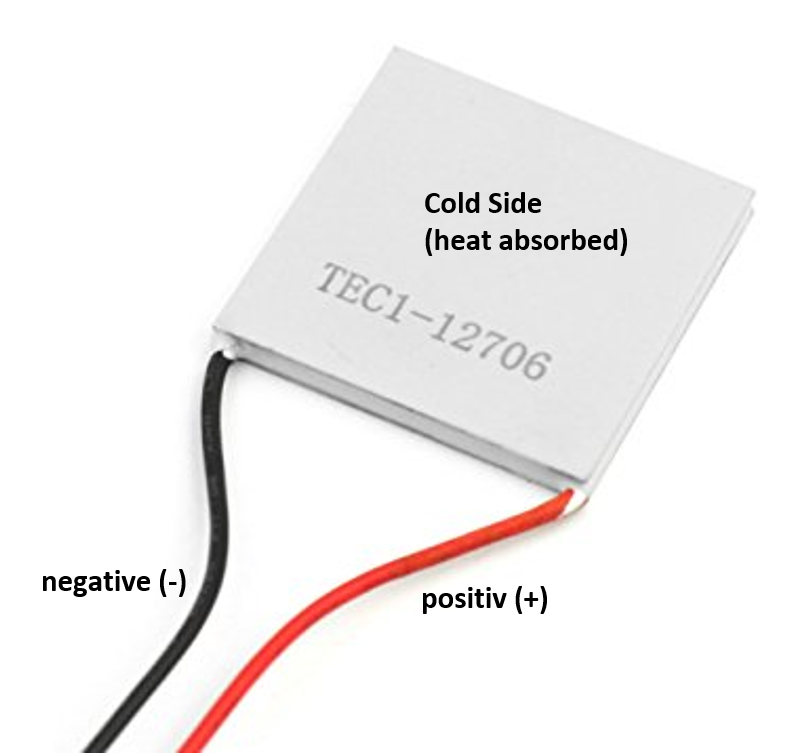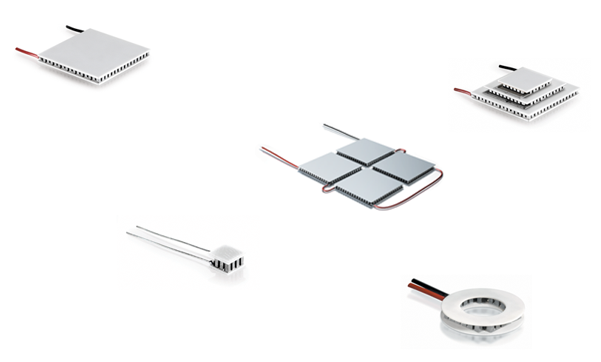Left side: Standard Peltier element      Right side: Special types of Peltier elements

Usually the manufacturer identification printed on the cold side of the Peltier element. This is the cold side if the positive supply voltage is connected with the red cable of the Peltier element

Since cables have a thermal capacity they are connected to the hot side of the Peltier element, not to decrease the cooling capacity of the element.

As you can see in the right picture, there are different types of Peltier elements.They vary in size and form, power and temperature range.

Sizes range: 1 mm x 1 mm up to 60 mm x 60 mm
Forms: Square, ring form, multistage, singlestage, sealed or non-sealed, custom forms
Temperature range: Temperature difference dTmax up to 130 °C (Multistage), max. temperature up to 200 °C
Maximum cooling capacity: up to 290 W

### Peltier Element Model

Peltier elements can be characterized by a model. The following three effects are subject to this model

• Peltier effect Qp: Heat transport from one side to the other. Described in this equation Qp = I * α * T
• Heat backflow QRth: Heat flow from the hot side to the cold side. Described in this equation QRth = dT / Rth
• Joule heating/losses QRv represent in the resistance Rv: Described in this equation QRv = I2 * Rv / 2.
The heat generated by Rv is equally divided between the hot and the cold side. The heat generated at the hot side is directly dissipated by the heatsink and is therefore not included in this equation.

The resulting pumped heat load Qc depends on the three effects Qp, QRth and QRv.

In the case of cooling, the equation for Qc. Is as follows: Qc = Qp - QRth - QRv.

### Parameters of a Peltier Element

Besides mechanical properties Peltier elements are characterized by four important parameters. Which are provided by the manufacturer: Qmax, dTmax, Umax, Imax

•  Qmax: Maximum heat pumping capacity at a temperature difference between the hot and cold side of 0 °K
•  dTmax: The maximum temperature difference across the Peltier element, when no heat is pumped
•  Imax: Current through the Peltier Element at  Qmax
•  Umax: Voltage through the Peltier Element at  Qmax

The parameters Qmax and dTmax are theoretical figures and they are used to describe the behavior of Peltier elements. However, those maximum values are never reached in a thermoelectric application. They are provided by the manufacturer to characterize the performance of the Peltier module.

In a thermoelectric application, there is always a trade-off between heat pump capacity Qc and temperature difference dT.

## Properties and behavior of Peltier elements

The following four diagrams characterize a Peltier element good. They are a useful to unterstand the properties and the behavior of Peltier elements. Similar diagrams are also used by manufacturers sometime, for example Ferrotec. The values in the charts are all relative.

### Heat Pumped vs. Current

This normalized diagram describes the relationship between the heat pump output on the y-axis and the current on the x-axis for different values of temperature differences between the hot and cold side (dT = Thot - Tcold) in the case of cooling.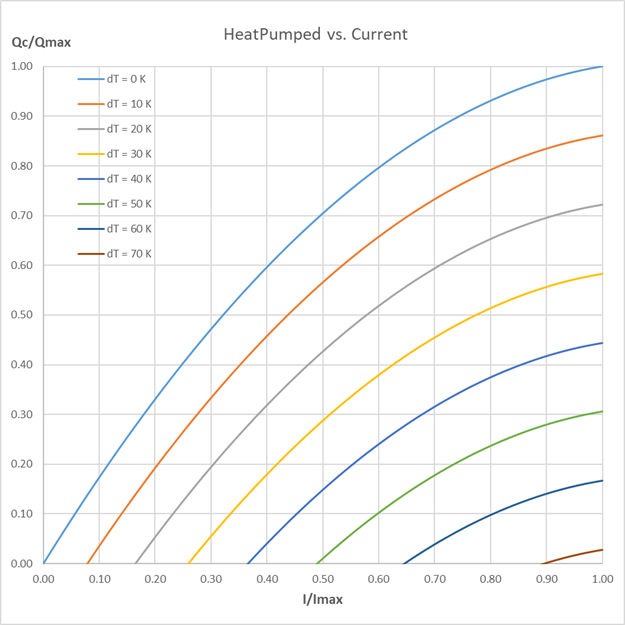Dynamics of a System. Normalized diagram Heat Pumped vs. Current

Only for relatively small temperature differences dT a significant amount of heat can be transferred. Multi-stage Peltier elements are used when higher temperature differences are needed.

The pumped heat QC and the temperature difference dT are inversely to each other, as heat is applied to the cold side the temperature difference is suppressed.

Normally, the current through for the Peltier element should be between 0 and 0.7 times Imax.

#### Dynamics of a System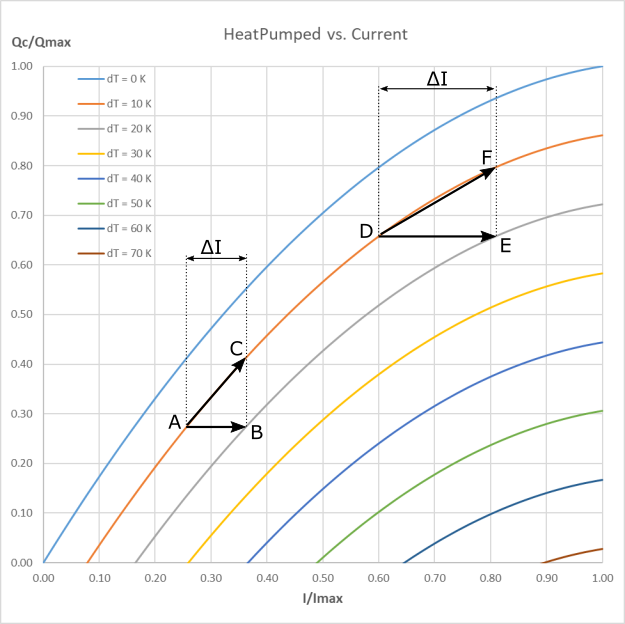Dynamics of a System. Normalized diagram Heat Pumped vs. Current

To understand the dynamics of the system, we can observe what happens if the temperature changes—and therefore the dT—or when the heat load is increased.

If we operate the Peltier element with a current around 25% of Imax it is possible to compensate a 10-Kelvin-rise of dT—point A to B—To ensure that the heat pump performance remains constant, the current must be increased. The heat pump capacity can be increased as well without changing dT, if we move from A to C.

If the working point is around 60% of Imax we need more current than in the previous example to compensate a 10-Kelvin-rise of dT—point D to E—when the heat pump capacity should stay unaffected. The heat pump capacity can still be increased without losing temperate difference, if we move from D to F.

However, if the Peltier element is operated near its maximum current, a change in temperature can’t be compensated by increasing the current. The transition from a lower to a higher temperature difference would result in a decrease of heat pump capacity.

### Coefficient of Performance (COP) (Efficiency)

The definition of the COP is the heat absorbed at the cold side QC divided by the input power Pel of the Peltier element: COP = QC / Pel. The COP is in principal the efficiency of the Peltier element when cooling.

The following diagram shows the performance (COP) versus the current ratio I / Imax, the values in this diagram are relative and normalized.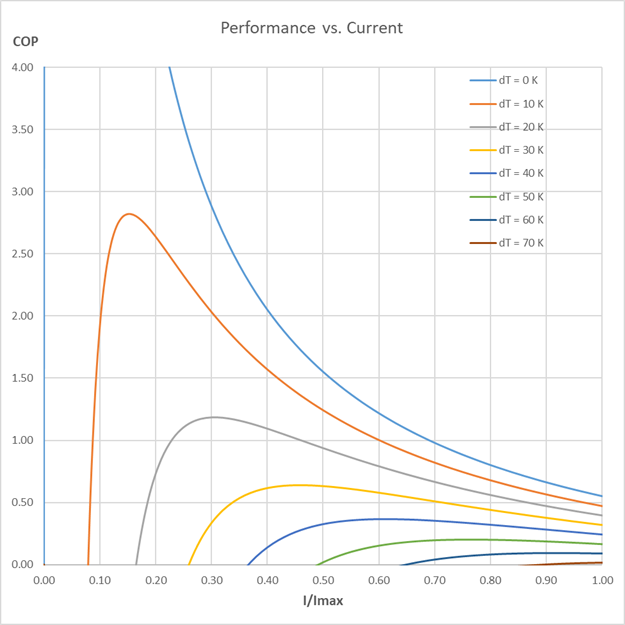This diagram shows the performance (COP) versus current relationship. Use it to find the operating current yielding the highest performance for a corresponding temperature difference dT.

On the left side, we see that the COP is maximum at the lowest temperature difference. Hence, we get a high amount of heat pumped per unit of electrical power. As we can see, depending on dT the corresponding COP maximum is at different current levels—with higher dT it moves to the right. If we follow the curve to the right, we find out that we must put a lot of electrical power into the system to get only a little heat pumped, what corresponds to a low COP value. We can also observe that higher currents are needed to produce higher temperature differences.

The reason why the COP does not start at zero with a dT > 0 K is because first the heat backflow QRth must be compensated by the Peltier effect Qp before the Peltier element cools.

### Heat Rejected of a Peltier Element

The following diagram shows the heat Qh to be dissipated on the warm side of the Peltier element versus current relationship when cooling.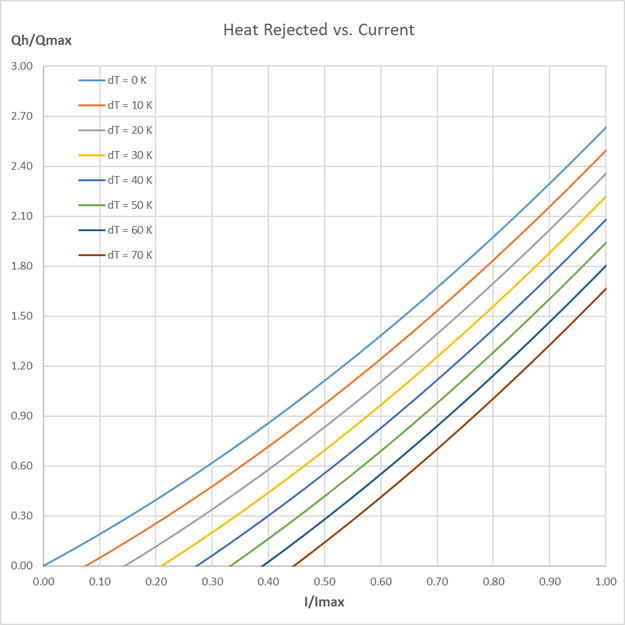Normalized diagram showing heat rejected by the heat sink versus current for various temperature differences dT.

The values are normalized and relative. As you can see Qh rejected by the Peltier element, can be up to 2.6 times Qmax. The amount of heat on the hot side Qh can be so large because the heat from the Peltier effect Qp and the heat of the loss resistance QRv must be dissipated. Qh = Qp + QRv applies.

### Dependency of Rejected Heat on dT

The following diagram shows the ratio between Qh and QC for different dT in case of cooling. The ratio Qh/ Qc is a factor how much more heat has to be dissipated on the hot side than on the cold side.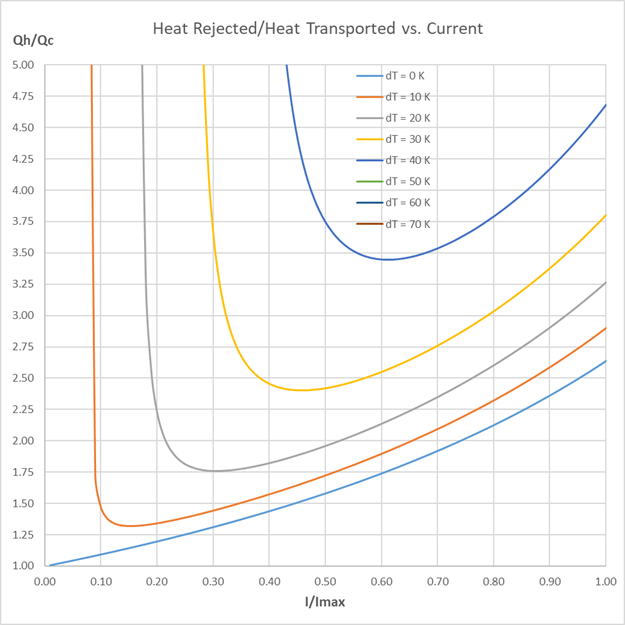Normalized diagram showing heat rejected by the heat sink per amount of heat pumped vs. current for different dT.

This means that for large dT, a large amount of heat is dissipated by the heat sink for a comparatively low amount of heat absorbed at the cold side of the Peltier element.
For example if you want to cool one watt on the cold side QC = 1 W. This results in a heat of 1.75 W on the hot side Qh = 1.75 W if the dt = 20 K. At dT = 40 K it is about 3.5 watts on the hot side Qh = 3.5 W.

### Voltage vs. Current

This normalized diagram describes the relationship between the voltage on the y-axis and the current on the x-axis for different values of temperature differences between the hot and cold side (dT = Thot - Tcold) in the case of cooling.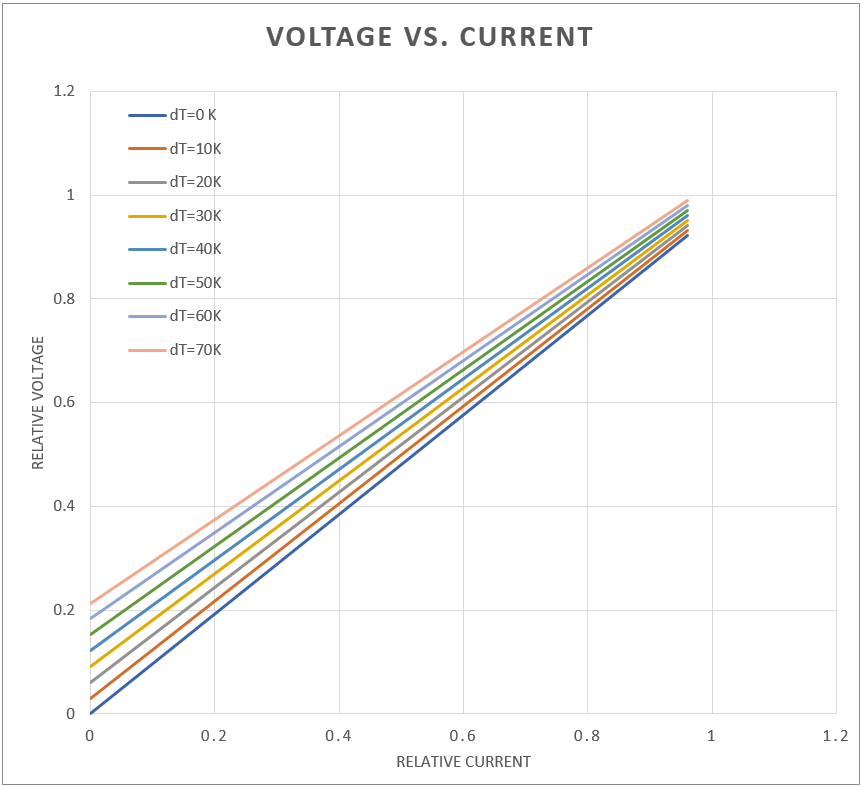Normalized diagram showing voltage vs. current for different dT.

As you can see, the curve is linear. The behavior of a Peltier element is the same as a resistor with a voltage source. The slope of the curve decreases with increasing dT. The displacement on the y-axis is related to the Seebeck effect.

### Multistage Peltier elements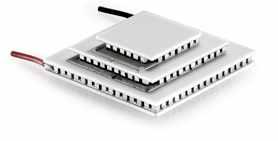Multistage Peltier element

All diagrams above apply to standard Peltier elements, but the behavior of Multistage Peltier elements is similar. Multistage Peltier elements are used when higher dT (up to 125 K) are required. But the Qmax is lower i.e. less heat can be dissipated. This is the disadvantage of Multistage Peltier elements.

## Manufacturers

 Manufacturer Description Country Deltron AGwww.deltron.ch Thermoelectric Modules Switzerland Ferrotecthermal.ferrotec.com Thermoelectric Modules USA, Asia, Europe RMT Ltd.www.rmtltd.ru Miniature to high power Peltier Elements Russia Lairdwww.lairdthermal.com Thermoelectric Modules United Kingdom II-VIwww.i-vi.com Thermoelectric Modules USA, Asia, Europe CUI Deviceswww.cuidevices.com Thermoelectric Modules USA Kryothermwww.kryothermtec.com Thermoelectric Modules Russia Peltron GmbHwww.peltier.de Thermoelectric Modules, Elements for Thermocycling Germany European Thermodynamics Ltdwww.europeanthermodynamics.com Thermoelectric Modules, Elements for Thermocycling Germany